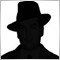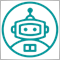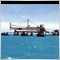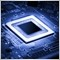• 概述
• 评论 (5)
• 评论 (1)
• 新特性

# MAPC

MAPC (Moving Average Percent's Change) shows the Moving Average's percent change from one period to another selected period allowing you to track the smoothed price change rate.

MAPC is calculated according to the following equation:

MAPC[i] = 100 * (MA[i] - MA[i - n]) / MA[i],

where:

• MA[i] - current Moving Average value;
• MA[i - n] - value of the Moving Average that is n periods away from the current Moving Average value.

### Input Parameters

• Period - Moving Average period; Method - smoothing method (Simple, Exponential, Smoothed, Linear weighted);
• Applied Price - applied price (Close, Open, High, Low, Median, Typical, Weighted);
• Displacement - shifting the Moving Average from one period to another selected period (Displacement = n in the above equation).

Note: if Displacement is less than 1 or more than Period, the following alert appears: "Incorrect Displacement value. Indicator will use value Displacement=Period".16101
Nikolay Gaylis 2019.01.14 08:2212010
2018.01.20 20:095698
2017.11.09 14:466186
2017.06.28 05:077170
2016.03.12 21:55

Improved stability and speed of the indicator.